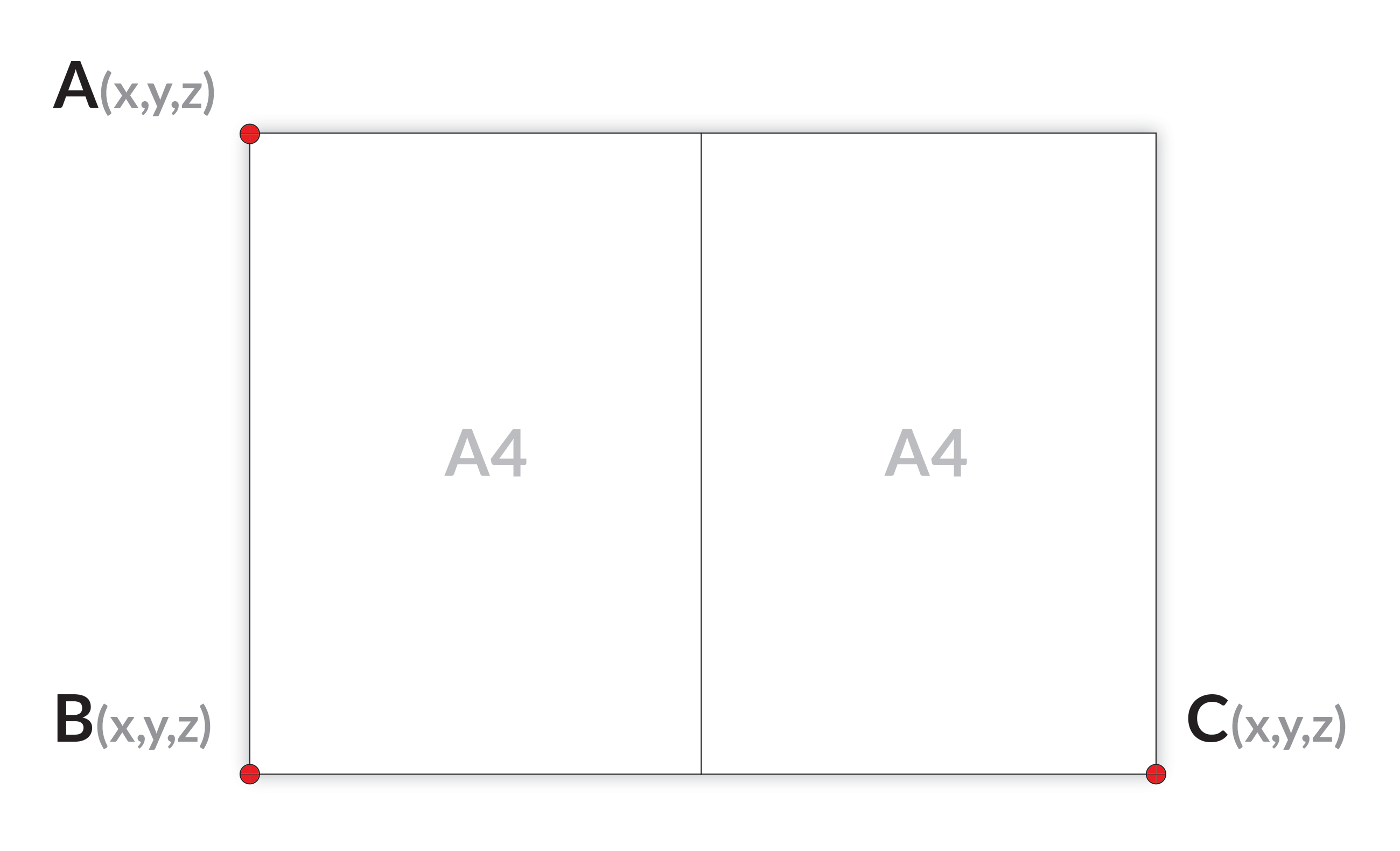# Robot accuracy¶

The limiting factor of robot accuracy is the robot trueness. Robot repeatability tends to be an order of magnitude better than their trueness. For example, it is typical to have a repeatability of 0.1 mm and a trueness of 1 mm. Therefore, this article focuses on robot trueness.

## What is robot trueness?¶

We define the robot trueness as the measure of how precisely a robot can move relative to a given point. That is, if you ask a robot to move 500 mm along the Y axis, will it actually move by 500 mm?

Robot trueness should not be confused with robot repeatability, which measures how consistently a robot can come back to a taught point.

Note

For blind applications, where the robot moves between fixed taught points, only the robot repeatability matters. For vision applications, both the robot repeatability and the robot trueness matter. Unfortunately, robot manufacturers usually only communicate robot repeatability in their datasheet and not robot trueness.

## Verifying the robot trueness¶

This section describes an easy way to verify the trueness of a robot by calculating the distance between three known points.

Warning

This verification is subject to manual error (jogging the robot to a given point). It is therefore not exact, but it can catch errors larger than 1 mm.

The idea of this test is to compare real and known distances with the distances perceived by the robot. For this, you need an object that contains distinctive points at which the robot can precisely point, and the distances between the points need to be exactly known. This could be corners of a table or corners of a sheet of paper. The robot needs to be able to reach all relevant points. Here we use two A4 sheets (equivalent to one A3 sheet) to illustrate the procedure.

In case of two A4 sheets make sure you attach them accurately and firm to each other as seen in the illustration below.Lay this paper in such a way that the corners are reachable by your robot.

The robot trueness depends on the robot pose. It is therefore recommended to measure the robot trueness inside the Region of Interest (ROI) of the application, where picking is meant to take place. For camera on robot, it is also interesting to verify the robot truenss at the detection pose(s).

Point your robot TCP exactly to points A, B and C, and write down the TCP coordinates given by the robot.

Tip

Make sure that the TCP orientation is kept the same for all points. The easiest is to have the TCP oriented at 0 degrees around X, Y and Z. This makes sure that eventual TCP errors are not affecting this test.

Calculate the distance between three corners by using this calculator.

The deviation from the expected distance indicates the trueness error of your robot.

For an A3 sheet of paper, the distances should be close to:

• A to B: 297 mm

• B to C: 420 mm

• A to C: 514.4 mm

## Correcting the robot trueness¶

If you observed unexpectedly high errors with your robot, contact your robot manufacturer. A new robot factory calibration might be required.

An industrial robot should typically be accurate to less than 1 mm.